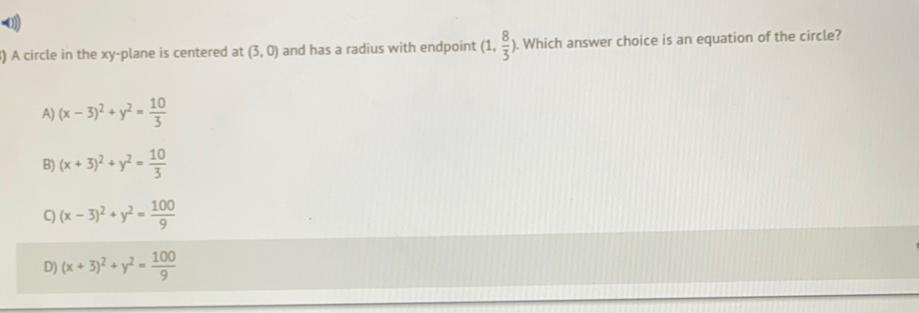Question:

# A circle in the xy-plane is centered at (3, 0) and has a radius with endpoint (1, 8/3). Which answer choice is an equation of the circle? A) (x – 3)^2 + y^2 = 10/3 B) (x + 3)^2 + y^2 =10/3 C)(x - 3)^A circle in the xy-plane is centered at (3, 0) and has a radius with endpoint (1, 8/3). Which answer choice is an equation of the circle? A) (x – 3)^2 + y^2 = 10/3 B) (x + 3)^2 + y^2 =10/3 C)(x - 3)^2 + y^2 =100/9 D) (x + 3)^2 + y^2 - 100/9